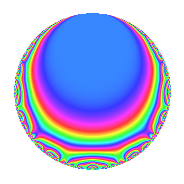Properties

 Label 2007.1.d.bLevel 2007 Weight 1 Character orbit 2007.d Self dual Yes Analytic conductor 1.002 Analytic rank 0 Dimension 3 Projective image $$D_{7}$$ CM disc. -223 Inner twists 2

Related objects

Newspace parameters

 Level: $$N$$ = $$2007 = 3^{2} \cdot 223$$ Weight: $$k$$ = $$1$$ Character orbit: $$[\chi]$$ = 2007.d (of order $$2$$ and degree $$1$$)

Newform invariants

 Self dual: Yes Analytic conductor: $$1.00162348035$$ Analytic rank: $$0$$ Dimension: $$3$$ Coefficient field: $$\Q(\zeta_{14})^+$$ Coefficient ring: $$\Z[a_1, a_2]$$ Coefficient ring index: $$1$$ Projective image $$D_{7}$$ Projective field Galois closure of 7.1.11089567.1

$q$-expansion

Coefficients of the $$q$$-expansion are expressed in terms of a basis $$1,\beta_1,\beta_2$$ for the coefficient ring described below. We also show the integral $$q$$-expansion of the trace form.

 $$f(q)$$ $$=$$ $$q$$ $$+ \beta_{1} q^{2}$$ $$+ ( 1 + \beta_{2} ) q^{4}$$ $$+ ( -1 + \beta_{1} - \beta_{2} ) q^{7}$$ $$+ ( 1 + \beta_{2} ) q^{8}$$ $$+O(q^{10})$$ $$q$$ $$+ \beta_{1} q^{2}$$ $$+ ( 1 + \beta_{2} ) q^{4}$$ $$+ ( -1 + \beta_{1} - \beta_{2} ) q^{7}$$ $$+ ( 1 + \beta_{2} ) q^{8}$$ $$+ ( 1 - \beta_{1} ) q^{14}$$ $$+ \beta_{1} q^{16}$$ $$-\beta_{2} q^{17}$$ $$+ \beta_{2} q^{19}$$ $$+ q^{25}$$ $$- q^{28}$$ $$+ \beta_{1} q^{29}$$ $$-\beta_{1} q^{31}$$ $$+ q^{32}$$ $$+ ( -1 - \beta_{2} ) q^{34}$$ $$+ ( -1 + \beta_{1} - \beta_{2} ) q^{37}$$ $$+ ( 1 + \beta_{2} ) q^{38}$$ $$+ ( 1 - \beta_{1} + \beta_{2} ) q^{41}$$ $$-\beta_{1} q^{43}$$ $$-\beta_{2} q^{47}$$ $$+ ( 1 - \beta_{1} ) q^{49}$$ $$+ \beta_{1} q^{50}$$ $$-\beta_{2} q^{53}$$ $$- q^{56}$$ $$+ ( 2 + \beta_{2} ) q^{58}$$ $$+ ( -2 - \beta_{2} ) q^{62}$$ $$+ ( -1 - \beta_{1} ) q^{68}$$ $$-\beta_{1} q^{73}$$ $$+ ( 1 - \beta_{1} ) q^{74}$$ $$+ ( 1 + \beta_{1} ) q^{76}$$ $$+ ( -1 + \beta_{1} ) q^{82}$$ $$+ \beta_{1} q^{83}$$ $$+ ( -2 - \beta_{2} ) q^{86}$$ $$+ ( 1 - \beta_{1} + \beta_{2} ) q^{89}$$ $$+ ( -1 - \beta_{2} ) q^{94}$$ $$+ ( -2 + \beta_{1} - \beta_{2} ) q^{98}$$ $$+O(q^{100})$$ $$\operatorname{Tr}(f)(q)$$ $$=$$ $$3q$$ $$\mathstrut +\mathstrut q^{2}$$ $$\mathstrut +\mathstrut 2q^{4}$$ $$\mathstrut -\mathstrut q^{7}$$ $$\mathstrut +\mathstrut 2q^{8}$$ $$\mathstrut +\mathstrut O(q^{10})$$ $$3q$$ $$\mathstrut +\mathstrut q^{2}$$ $$\mathstrut +\mathstrut 2q^{4}$$ $$\mathstrut -\mathstrut q^{7}$$ $$\mathstrut +\mathstrut 2q^{8}$$ $$\mathstrut +\mathstrut 2q^{14}$$ $$\mathstrut +\mathstrut q^{16}$$ $$\mathstrut +\mathstrut q^{17}$$ $$\mathstrut -\mathstrut q^{19}$$ $$\mathstrut +\mathstrut 3q^{25}$$ $$\mathstrut -\mathstrut 3q^{28}$$ $$\mathstrut +\mathstrut q^{29}$$ $$\mathstrut -\mathstrut q^{31}$$ $$\mathstrut +\mathstrut 3q^{32}$$ $$\mathstrut -\mathstrut 2q^{34}$$ $$\mathstrut -\mathstrut q^{37}$$ $$\mathstrut +\mathstrut 2q^{38}$$ $$\mathstrut +\mathstrut q^{41}$$ $$\mathstrut -\mathstrut q^{43}$$ $$\mathstrut +\mathstrut q^{47}$$ $$\mathstrut +\mathstrut 2q^{49}$$ $$\mathstrut +\mathstrut q^{50}$$ $$\mathstrut +\mathstrut q^{53}$$ $$\mathstrut -\mathstrut 3q^{56}$$ $$\mathstrut +\mathstrut 5q^{58}$$ $$\mathstrut -\mathstrut 5q^{62}$$ $$\mathstrut -\mathstrut 4q^{68}$$ $$\mathstrut -\mathstrut q^{73}$$ $$\mathstrut +\mathstrut 2q^{74}$$ $$\mathstrut +\mathstrut 4q^{76}$$ $$\mathstrut -\mathstrut 2q^{82}$$ $$\mathstrut +\mathstrut q^{83}$$ $$\mathstrut -\mathstrut 5q^{86}$$ $$\mathstrut +\mathstrut q^{89}$$ $$\mathstrut -\mathstrut 2q^{94}$$ $$\mathstrut -\mathstrut 4q^{98}$$ $$\mathstrut +\mathstrut O(q^{100})$$

Basis of coefficient ring in terms of $$\nu = \zeta_{14} + \zeta_{14}^{-1}$$:

 $$\beta_{0}$$ $$=$$ $$1$$ $$\beta_{1}$$ $$=$$ $$\nu$$ $$\beta_{2}$$ $$=$$ $$\nu^{2} - 2$$
 $$1$$ $$=$$ $$\beta_0$$ $$\nu$$ $$=$$ $$\beta_{1}$$ $$\nu^{2}$$ $$=$$ $$\beta_{2}\mathstrut +\mathstrut$$ $$2$$

Character Values

We give the values of $$\chi$$ on generators for $$\left(\mathbb{Z}/2007\mathbb{Z}\right)^\times$$.

 $$n$$ $$226$$ $$893$$ $$\chi(n)$$ $$-1$$ $$1$$

Embeddings

For each embedding $$\iota_m$$ of the coefficient field, the values $$\iota_m(a_n)$$ are shown below.

For more information on an embedded modular form you can click on its label.

Label $$\iota_m(\nu)$$ $$a_{2}$$ $$a_{3}$$ $$a_{4}$$ $$a_{5}$$ $$a_{6}$$ $$a_{7}$$ $$a_{8}$$ $$a_{9}$$ $$a_{10}$$
1783.1
 −1.24698 0.445042 1.80194
−1.24698 0 0.554958 0 0 −1.80194 0.554958 0 0
1783.2 0.445042 0 −0.801938 0 0 1.24698 −0.801938 0 0
1783.3 1.80194 0 2.24698 0 0 −0.445042 2.24698 0 0
 $$n$$: e.g. 2-40 or 990-1000 Significant digits: Format: Complex embeddings Normalized embeddings Satake parameters Satake angles

Inner twists

Char. orbit Parity Mult. Self Twist Proved
1.a Even 1 trivial yes
223.b Odd 1 CM by $$\Q(\sqrt{-223})$$ yes

Hecke kernels

This newform can be constructed as the kernel of the linear operator $$T_{2}^{3}$$ $$\mathstrut -\mathstrut T_{2}^{2}$$ $$\mathstrut -\mathstrut 2 T_{2}$$ $$\mathstrut +\mathstrut 1$$ acting on $$S_{1}^{\mathrm{new}}(2007, [\chi])$$.